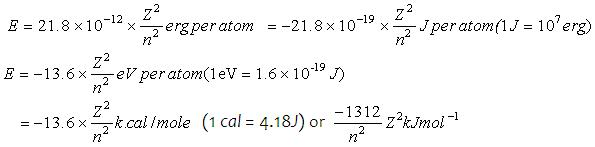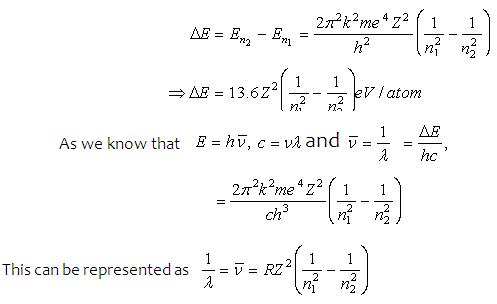1800-1023-196

+91-120-4616500

# Advantages of Bohr’s Theory

## Advantages of Bohr’s Theory

(i) Bohr’s theory satisfactorily explains the spectra of species having one electron, viz. hydrogen atom, He+, Li2+ etc.
(ii) Calculation of radius of Bohr’s orbit: According to Bohr, radius of nth orbit in which electron moves is rn = [h2/4π2me2k] n2/Z

Where, n = Orbit number, m = Mass number [9.1×10-31kg],e = Charge on the electron [1.6×10-19] Z = Atomic number of element, k = Coulombic constant [9×109Nm2c-2] After putting the values of m,e,k,h, we get. rn = n2/Z×0.529Å

(iii) Calculation of velocity of electron Vn = 2πe2ZK/nh, Vn = [Ze2/mr]1/2; Vn = 2.188×108Z/n (cm.sec-1
(iv) Calculation of energy of electron in Bohr’s orbit

Total energy of electron = K.E. + P.E. of electron = k Ze2/2r - kZe2/r = kZe2/2r

Substituting of r, gives us E = (-2π2mZ2e4k2/n2h2) Where, n=1, 2, 3……….∞

Putting the value of m, e, k, h, π we getWhen an electron jumps from an outer orbit (higher energy) n2 to an inner orbit (lower energy)n1, then the energy emitted in form of radiation is given byWhere, R = 2π2K2me4/ch3 R is known as Rydberg constant. Its value to be used is 109678cm-1

The negative sign in the above equations shows that the electron and nucleus form a bound system, i.e., the electron is attracted towards the nucleus. Thus, if electron is to be taken away from the nucleus, energy has to be supplied. The energy of the electron in n = 1 orbit is called the ground state energy; that in the n = 2 orbit is called the first excited state energy, etc. When n = ∞ then E = 0 which corresponds to ionized atom i.e., the electron and nucleus are infinitely separated H → H+ + e- (ionization).

## NEET & AIIMS Exam Sample Papers

 AIIMS SAMPLE PAPERS View More NEET SAMPLE PAPERS View More